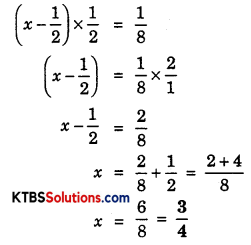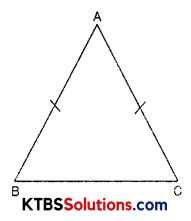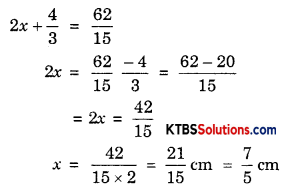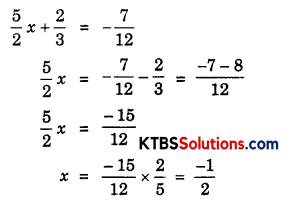# KSEEB Solutions for Class 8 Maths Chapter 2 Linear Equations in One Variable Ex 2.2

You can Download KSEEB Solutions for Class 8 Maths Chapter 2 Linear Equations in One Variable Ex 2.2 Questions and Answers helps you to revise the complete syllabus.

## KSEEB Solutions for Class 8 Maths Chapter 2 Linear Equations in One Variable Ex 2.2

Question 1.
If you subtract $$\frac{1}{2}$$ from a number and multiply the result by $$\frac{1}{2}$$, you get $$\frac{1}{8}$$. What is the number?
Solution:
Let the required number = x
Subtracting $$\frac{1}{2}$$ from the number, we get = (x – $$\frac{1}{2}$$)
According to the question,The required number is $$\frac{3}{4}$$.

Question 2.
The perimeter of a rectangular swimming pool is 154 m. Its length is 2 m more than twice its breadth. What are the length and the breadth of the pool?
Solution:
Let the breadth of swimming pool = x mts
Length of pool = (2x + 2) mts
Perimeter of rectangle = 2(l + b)
= 2 [(2x + 2) + x]
= 2 [2x + 2 + x]
= 2[3x + 2] mts
Perimeter of rectangular swimming pool = 154
⇒ 2 (3x + 2) = 154
⇒ 3x + 2 = $$\frac{154}{2}$$
⇒ 3x + 2 = 77
⇒ 3x = 77 – 2
⇒ 3x = 75
⇒ x = 25
Length = 2 × 25 + 2 = 50 + 2 = 52 mQuestion 3.
The base of an isosceles triangle is $$\frac{4}{3}$$ cm. The perimeter of the triangle is 4$$\frac{2}{15}$$ cm. What is the length of either of the remaining equal sides?
Solution:Let the equal sides of an isosceles triangle be AB = AC
Let equal sides = x cm
Base BC = $$\frac{4}{3}$$ cm
Perimeter of ΔABC = AB + BC + AC
= x + $$\frac{4}{3}$$ + x
= (2x + $$\frac{4}{3}$$) cm
But Perimeter of ΔABC = 4$$\frac{2}{15}$$ = $$\frac{62}{15}$$ cmHence, each equal sides is $$\frac{7}{5}$$ cm

Question 4.
Sum of two numbers is 95. If one exceeds the other by 15, find the numbers.
Solution:
Let one number = x
2nd number = (x + 15)
According to question,
x + (x + 15) = 95
⇒ x + x + 15 = 95
⇒ 2x + 15 = 95
⇒ 2x = 95 – 15
⇒ 2x = 80
⇒ x = 40
(x + 15) = 40 + 15 = 55
Hence, numbers are 40, 55.

Question 5.
Two numbers are in the ratio 5 : 3. If they differ by 18, what are the numbers?
Solution:
Let the ratio be x
The numbers be 5x and 3x
5x – 3x = 18
⇒ 2x = 18
⇒ x = 9
Numbers are 5x = 5 × 9 = 45
3x = 3 × 9 = 27Question 6.
Three consecutive integers add up to 51. What are these integers?
Solution:
Let first integer = x
Second integer = (x + 1)
Third integer = (x + 2)
According to question,
x + x + 1 + x + 2 = 51
⇒ 3x + 3 = 51
⇒ 3x = 51 – 3
⇒ 3x = 48
⇒ x = 16
First integer = 16
Second integer = 16 + 1 = 17
Third integer = 16 + 2 = 18

Question 7.
The sum of three consecutive multiples of 8 is 888. Find the multiples.
Solution:
Let First multiple of 8 be = 8x
Second multiple be = (8x + 8)
Third multiple be = 8x + 8 + 8 = 8x + 16
According to question,
8x + 8x + 8 + 8x + 16 = 888
24x + 24 = 888
24x = 888 – 24
24x = 864
x = 36
8x = 8 × 36 = 288
8x + 8 = 288 + 8 = 296
8x + 16 = 8 × 36 + 16
= 288 + 16
= 304
The numbers are 288, 296, 304.

Question 8.
Three consecutive integers are such that when they are taken in increasing order and multiplied by 2, 3, and 4, respectively, they add up to 74. Find these numbers.
Solution:
Let First integer = x
Second integer = (x + 1)
Third integer = (x + 2)
Since, first integer is multiple of 2
∴ 2 is factor of x
Hence, number = 2x
Second number = 3(x + 1)
Third number = 4(x + 2)
According to question,
2x + 3(x + 1) + 4(x + 2) = 74
⇒ 2x + 3x + 3 + 4x + 8 = 74
⇒ 9x + 11 = 74
⇒ 9x = 74 – 11
⇒ 9x = 63
⇒ x = 7
First integer = 7
Second integer = (7 + 1) = 8
Third integer = (7 + 2) = 9Question 9.
The ages of Rahul and Haroon are in the ratio 5 : 7. Four years later the sum of their ages will be 56 years. What are their present ages?
Solution:
Let the ratio be x
Rahul’s present age = 5x yrs
Haroon’s present age = 7x yrs
After 4 years,
Rahul’s age = (5x + 4) yrs
Haroon’s age = (7x + 4) yrs
According to question,
(5x + 4) + (7x + 4) = 56
⇒ 5x + 4 + 7x + 4 = 56
⇒ 12x + 8 = 56
⇒ 12x = 56 – 8
⇒ 12x = 48
⇒ x = 4
Rahul’s present age = 5 × 4 = 20 years
Haroon’s present age = 7 × 4 = 28 years

Question 10.
The number of boys and girls in a class is in the ratio 7 : 5. The number of boys is 8 more than the number of girls. What is the total class strength?
Solution:
Let the ratio be = x
The number of boys = 7x
Number of girls = 5x
According to question,
7x – 5x = 8
⇒ 2x = 8
⇒ x = 4
Number of boys = 7 × 4 = 28
Number of girls = 5 × 4 = 20
Total strength of class = 28 + 20 = 48Question 11.
Baichung’s father is 26 years younger than Baichung’s grandfather and 29 years older than Baichung. The sum of the ages of all three is 135 years. What is the age of each one of them?
Solution:
Let age of Baichung = x years
Baichung’s father age = (x + 29) years
Baichung’s grandfather’s age = (x + 29 + 26) = (x + 55) years
According to question,
x + x + 29 + x + 55 = 135
⇒ 3x + 84 = 135
⇒ 3x = 135 – 84
⇒ 3x = 51
⇒ x = 17
Baichung’s age = 17 years
Father’s age = 17 + 29 = 46 years
Grandfather’s age = 17 + 55 = 72 years

Question 12.
Fifteen years from now Ravi’s age will be four times his present age. What is Ravi’s present age?
Solution:
Let present age of Ravi = x years
15 years later Ravi’s age = (x + 15) years
According to question,
4x = (x + 15)
⇒ 4x – x = 15
⇒ 3x = 15
⇒ x = 5
Ravi’s age = 5 years.

Question 13.
A rational number is such that when you multiply it by $$\frac{5}{2}$$ and add $$\frac{2}{3}$$ to the product, you get $$-\frac{7}{12}$$. What is the number?
Solution:
Let the number be x
When x is multiplied by $$\frac{5}{2}$$ = $$\frac{5}{2} x$$
According to question,Rational number = $$\frac{-1}{2}$$

Question 14.
Lakshmi is a cashier in a bank. She has currency notes of denominations Rs. 100, Rs. 50 and Rs. 10, respectively. The ratio of the number of these notes is 2 : 3 : 5. The total cash with Lakshmi is Rs. 4,00,000. How many notes of each denomination does she have?
Solution:
Let the ratio = x
Rs. 100, Rs. 50 and Rs. 10 ratio = 2 : 3 : 5
Number of Rs. 100 notes = 2x
Number of Rs. 50 notes = 3x
Number of Rs. 10 notes = 5x
Value of Rs. 100 notes = 100 × 2x = 200x
Value of Rs. 50 notes = 50 × 3x = 150x
Value of Rs. 10 notes = 10 × 5x = 50x
According to question,
200x + 150x + 50x = 4,00,000
⇒ 400x = 4,00,000
⇒ x = $$\frac{4,00,000}{400}$$ = 1000
Number of Rs. 100 notes = 1000 × 2 = 2000
Number of Rs. 50 notes = 1000 × 3 = 3000
Number of Rs. 10 notes = 1000 × 5 = 5000Question 15.
I have a total of Rs. 300 in coins of denomination Re. 1, Rs. 2, and Rs. 5. The number of Rs. 2 coins is 3 times the number of Rs. 5 coins. The total number of coins is 160. How many coins of each denomination are with me?
Solution:
Total number of coins = 160
Let Re. 1 coins = x
Rs. 2 coins = y
Rs. 5 coins = z
Number of Rs. 2 coin = 3 × Number of Rs. 5 coin
y = 3z
According to question,
x + y + z = 160
⇒ x + 3z + z = 160
⇒ x + 4z = 160 ………. (1)
Value of Re. 1 coin = 1 × x = x
Value of Rs. 2 coin = 2y = 2y
Value of Rs. 5 coin = 5z = 5z
x + 2y + 3z = 300
⇒ x + 2 (3z) + 5z = 300
⇒ x + 6z + 5z = 300
⇒ x + 11z = 300
⇒ x = (300 – 11z) ……….. (2)
Substituting the value of x in equation 1
300 – 11z + 4z = 160
⇒ 300 – 7z = 160
⇒ 300 – 160 = 7z
⇒ 140 = 7z
⇒ z = 20
y = 3z = 3 × 20 = 60
x + y + z = 160
⇒ x + 20 + 60 = 160
⇒ x = 160 – 80
⇒ x = 80
Hence, Re. 1 coins = 80
Rs. 2 coins = 60
Rs. 5 coins = 20Question 16.
The organisers of an essay competition decide that a winner in the competition gets a prize of Rs. 100 and a participant who does not win gets a prize of Rs. 25. The total prize money distributed is Rs. 3,000. Find the number of winners, if the total number of participants is 63.
Solution:
Total number of participants = 63
Let number of winners = x
Number who does not win = (63 – x)
Prize for each winner = Rs. 100
Prize for non-winner = Rs. 25
According to question,
100x + 25 (63 – x) = 3000
⇒ 100x + 1575 – 25x = 3000
⇒ 75x + 1575 = 3000
⇒ 75x = 3000 – 1575
⇒ 75x = 1425
⇒ x = 19
Number of winners = 19